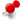# Confusion about any changes to the Transform3d Matrix4f or Matrifx4D.ClassicListThreaded7 messagesOpen this post in threaded view
|

## Confusion about any changes to the Transform3d Matrix4f or Matrifx4D.

 Until now, I had been under the impression that a Transform3D what composed of a start Matrix, which was 3x3 and located on the upper left, with modes bits for them in 4th row, and that the "result" column was the fourth column. -Yet having read jogamp java3D 1.6 javadoc for the Transform3D class, it appears that the result column is no longer the 4th one but is the 1st one. -It also now appears that the 3x3 values are additions, and not multiples (?)Can someone tell me what the true story is for my two dash points, as well as just tell me where I can get the true story for the Jogamp Java3D 1.6 Transform3d 4x4 matrix?  Is this matrix the same or different as the one needed for the JavaFX Transform class?
Open this post in threaded view
|

## Re: Confusion about any changes to the Transform3d Matrix4f or Matrifx4D.

Open this post in threaded view
|

## Re: Confusion about any changes to the Transform3d Matrix4f or Matrifx4D.

 All of this effort is really excellent, and thank you. Matrix m4 makes it clear.  The internal 3x3 Matrix is always upper left.  The point/vector mode setting for each 3D vector in the 3x3 matrix is in the 4th row beneath them.  The destination/conclusion vector is clearly in the 4th column.  All of which is the 4x4 format. -How was m6 acheived?  Rotation Matrices need to multiply from the left of the matrix they aim to affect, in Mathematics (Linear Algebra).   What was the Rotation Matrix Used?  What are the Row and Column count of the rotation matrix used to acheive m6?
Open this post in threaded view
|

## Re: Confusion about any changes to the Transform3d Matrix4f or Matrifx4D.

 m6 is a multiplication of 2 matrix4fs. Firstly note the Transfrom3D is just a convenience wrapper around a matrix 4x4, it supplies lots of helper functions and can be given to TransformGroups. (Note note it's actually wrapping a column-major double which can be thought of as a matrix 4x4) For m6 I creates a rotation matrix (in t2) which was a PI rotation (180 degrees) around the X axis (this constructed in the AxisAngle4f(x,y,z,rot)). Then I constructed a translation matrix (in t) of 10 in +Y ( using the setTranslation()) The I set t2 to be t2*t (in the line t2.mul(t) ) which is exactly the same as multiplying the 2 internal matrices together, then I wrote out the resulting matrix showing the upper 3x3 rotation and the translation column. In all cases the Transform3D hold 4x4 matrices, and although Matrix3f exists it is very rare to use it in Java3D because it is ambiguous as to whether you are attempting full 2d or rotational 3d maths. I believe this is a key difference to JavaFX where the upper 3x3 can be used either way depending on the domain (2d or 3d). Finally as you can tell from my simple words and trivial examples I'm no expert in matrix maths and it looks like you are well ahead of me.
Open this post in threaded view
|

## Re: Confusion about any changes to the Transform3d Matrix4f or Matrifx4D.

 -Could you type in values for the matrix m6 in a reply here, please? -In terms of the position of the 3x3 matrix in the 4x4 one, is the 3x3 upper left or upper right?